# 1 Introduction, the R environment, and the Data Example

Designs that are called “Completely Randomized Factorial Designs”, presume assignment of cases to groups independently. They are sometimes called “between subjects” designs in the psychological sciences. Two independent variables are factorially arranged so that each level of each factor is found in combination with each level of the other factor.

The goal of this document is to provide a fairly comprehensive overview of techniques to obtain all of the core assessments and tests that a researcher would typically need to employ. This includes assessment of the”omnibus” main effects and interactions. It also includes coverage of simple main effects. In addition, the ability to employ analytical/orthogonal conrasts is a central part of the analysis. Contrasts on all main effects, interactions, and simple main effects are all possible in R. Several post hoc, pairwise comparison, approaches are also demonstrated.

Additional sections cover effect size computations, graphical exploration and presentation of the data, assessment of assumptions, and some robust methods.

One issue with programming software to handle these designs is the non-uniformity of the language used to describe effects. In particular the phrases “simple main effect”, “simple main effect contrasts”, “partial interactions”, “interaction contrasts” and “interaction comparisons” do not gain usage much beyond the experimental design textbooks - particularly those from the behavioral sciences such as Winer, Kirk, Keppel, etc. In R, these effects are, with some labor, possible to define and test, but the labels are not those we are comfortable with. Newer add-on packages are being refined to make evaluation of these followups to the omnibus tests more approachable. The user should not expect the approach to be as direct/simple as it was with our consideration of the SPSS MANOVA procedure.

The document is constantly under revision as additional methods become available in new R packages or R packages that I learn about.

Resampling methods such as bootstrapping are not included at this time and will be covered in a separate document.

## 1.1 First, load the packages that will be required

Quite a few packages are used in this document. In many code chunks, where a the package origin of a function is not described or obvious, I use the `pkgname::functionname` format when a function is called. For example `psych::describeBy` calls the `describeBy function from the **psych** package. This format is not used when functions come from base system packages. One can always ask for help on a function (`?functionname`) to establish the package of its origin if I’ve been inconsistent in this formatting.

``````library(psych,quietly=TRUE, warn.conflicts=FALSE)
library(car,quietly=TRUE, warn.conflicts=FALSE)
library(foreign,quietly=TRUE, warn.conflicts=FALSE)
library(ggplot2,quietly=TRUE, warn.conflicts=FALSE)
library(ggthemes,quietly=TRUE, warn.conflicts=FALSE)
library(phia,quietly=TRUE, warn.conflicts=FALSE)
library(plyr,quietly=TRUE, warn.conflicts=FALSE)
library(ez,quietly=TRUE, warn.conflicts=FALSE)
library(sciplot,quietly=TRUE, warn.conflicts=FALSE)
library(granova,quietly=TRUE, warn.conflicts=FALSE)
library(afex,quietly=TRUE, warn.conflicts=FALSE)
library(emmeans,quietly=TRUE, warn.conflicts=FALSE)
library(sjstats,quietly=TRUE, warn.conflicts=FALSE)
library(effectsize,quietly=TRUE, warn.conflicts=FALSE)
library(knitr,quietly=TRUE, warn.conflicts=FALSE)
#library(kableExtra,quietly=TRUE, warn.conflicts=FALSE)
library(gt,quietly=TRUE, warn.conflicts=FALSE)
library(nortest,quietly=TRUE, warn.conflicts=FALSE)
library(BayesFactor,quietly=TRUE, warn.conflicts=FALSE)
library(Rmisc,quietly=TRUE, warn.conflicts=FALSE)``````

## 1.2 Obtain the Data

The data set used in this document is the 3x2 factorial employed for several prior illustrations in the 510/511 sequence. It was first introduced as the “example1” data set in the fall. The DV was the number of words recalled in a memorized list. IV’s were a treatment variable where participants received either positive or negative feedback during acquisition or were in a control condition. The second IV was an age categorization of young vs older participants. I believe that this data set originally came from an early edition of the Keppel experimental design textbook (perhaps Keppel and Zedeck).

Note that if we imported via the .csv file, R would have changed the order of the Praise/Reproof/None categories to alphabetical. The read.SPSS function we used (from the foreign package) permits us to keep the order as we used them in SPSS. This is very helpful for the sake of comparison to our earlier SPSS work. Of course, we could do a rework of the order in R, but this way is faster.

The example is an “equal N” design, so we will not address issues of Type I/II/III SS in this document.

Note that the ‘attach’ function is used so that variables may be simply named later in the document without the ‘\$’ convention.

``````#prn.data <- read.spss(file.choose(), use.value.labels=TRUE,
#                      max.value.labels=Inf, to.data.frame=TRUE)
max.value.labels=Inf, to.data.frame=TRUE)``````
``## re-encoding from CP1252``
``````#prn.data <- read.csv("2way_base.csv", stringsAsFactors=TRUE)
attach(prn.data)
# kable is used to provide nicer formatting of this table;  the 'headTail' function is useful
#    kable_styling(full_width = F, position = "left")
``````##      group      age errors
## 1   PRAISE CHILDREN      4
## 2   PRAISE CHILDREN      7
## 3   PRAISE CHILDREN      5
## 4   PRAISE CHILDREN      3
## ...   <NA>     <NA>    ...

# 2 Descriptive Statistics

Exploratory data analsis is always important. The approach taken here is more or less the standard sequence we have used for other illustrations.

## 2.1 Numerical summaries of the DV, by group

Using the psych package, look at some descriptive statistics. Note that type=2 sets the type of skewness and kurtosis coefficients used in the `describeBy` function

``````library(psych)
db1 <- describeBy(errors,list(group,age),mat=TRUE,type=2, digits=3)
# I subset the db1 data frame to leave out some of the descriptive stats
db1[,c(1:3, 5:8,11:16)]``````

Or use `gt' or knitr::kable` with `kableExtra::kable_styling` to produce a more nicely formatted table:

``````# kable(db1[,c(2:3, 5:8,11:16)]) %>%
#     kable_styling(full_width = F, position = "left")
gt(db1[,c(2:3, 5:8,11:16)])``````
group1 group2 n mean sd median min max range skew kurtosis se
PRAISE CHILDREN 7 5.000 1.414 5 3 7 4 0.000 -1.200 0.535
REPROOF CHILDREN 7 8.714 1.113 9 7 10 3 -0.249 -0.944 0.421
NONE CHILDREN 7 6.429 1.512 7 4 8 4 -0.620 -0.809 0.571
PRAISE ADULTS 7 5.429 1.718 6 2 7 5 -1.487 2.666 0.649
REPROOF ADULTS 7 2.857 1.345 3 1 5 4 0.352 -0.302 0.508
NONE ADULTS 7 5.000 2.000 4 3 8 5 0.525 -1.550 0.756

## 2.2 Data Exploration via Graphical Display

Several types of graphical displays are illustrated here to provide a rapid visual impression of the data set.

### 2.2.1 Stiprcharts/DotPlots

It is possible to use the ‘stripchart’ function the way we used it for a 1-way design, by passing the factorial nature of this 2way in the model formula. The product may be a useful EDA method, but it doesn’t label the Y axis completely with my bare-bones example. Using this may require exploring control of graph attributes a bit more, especially if there are more than 3 and 2 levels of the factors.

``stripchart(errors~group*age, method="stack")``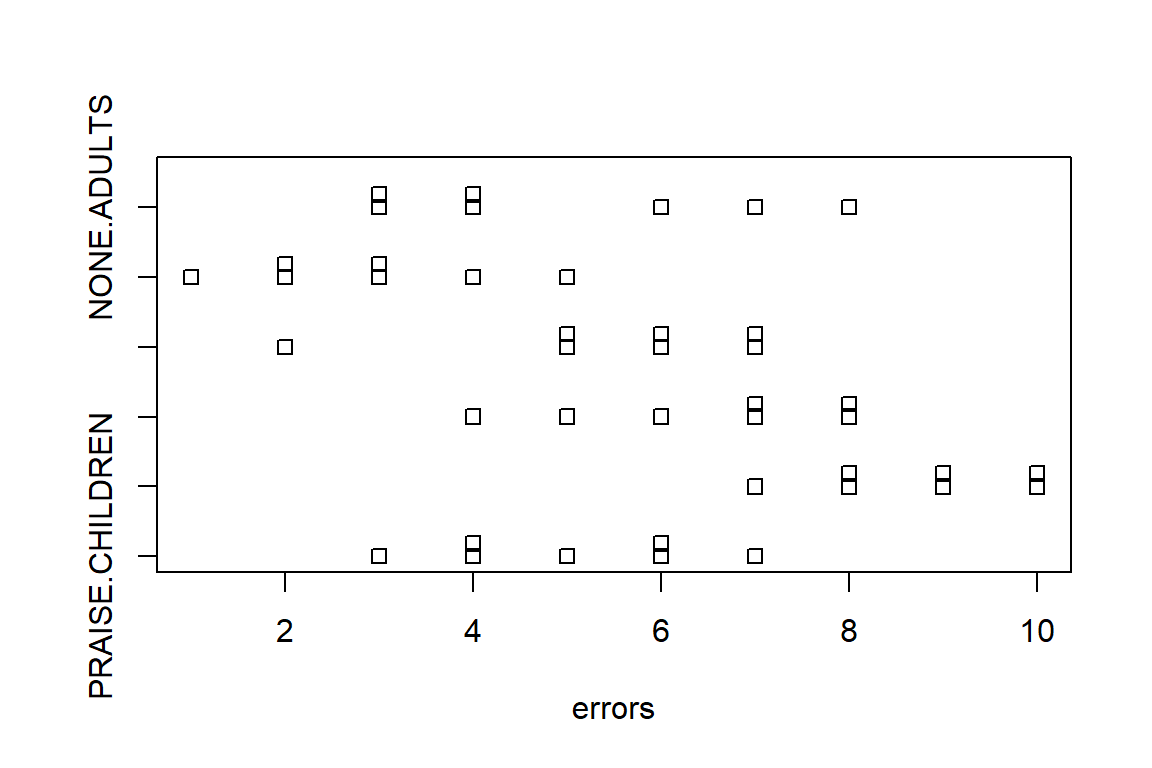### 2.2.2 Main effect variation

The ‘plot.design’ function from the base R installation provides a quick way to “see” main effect variation.

``````#win.graph() # or x11() or quartz()
plot.design(errors~age*group, main="Examine Main Effects")``````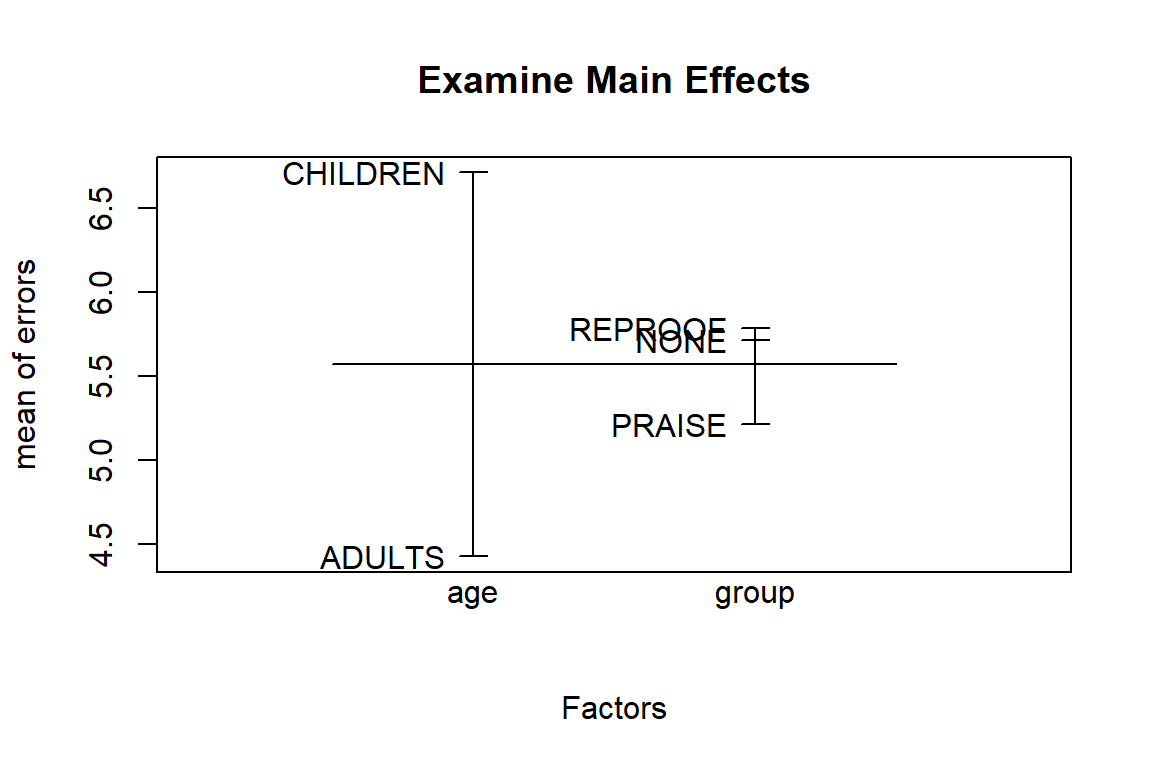### 2.2.3 Distributions within the groups

We may want to examine the distributon of the DV within each of the six groups. I’ve done this here by producing a ggplot2 graph that creates “facets” for the groups. For our data set, with such a low N, the frequency histograms may not be very helpful above and beyond what simple dot plots show us.

``````# first without kernel densities
p1a <- ggplot(prn.data, aes(errors))
p1b <- p1a + geom_histogram(
binwidth=1,
col="black",
fill="grey80") +
facet_wrap(age~group)
p1c <- p1b +theme_bw()
#win.graph() # or x11() or quartz()
#p1c
#now with kernel densities
p2a <- ggplot(prn.data, aes(errors))
p2b <- p2a + geom_histogram(aes(y =..density..),
binwidth=1,
col="black",
fill="grey80") +
geom_density(col="black") +
facet_wrap(age~group)
p2c <- p2b +theme_bw()
#win.graph() # or x11() or quartz()
p2c``````
``````## Warning: The dot-dot notation (`..density..`) was deprecated in ggplot2 3.4.0.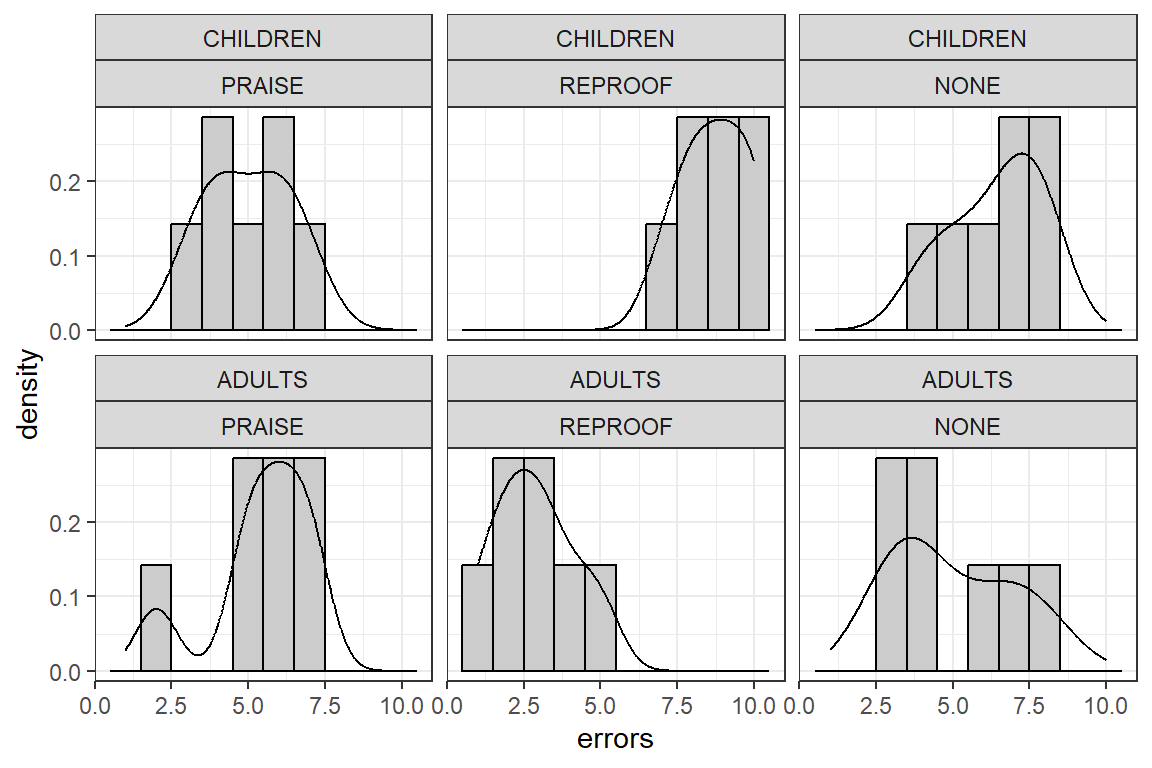### 2.2.4 Clustered Boxplots

The base system ’boxplot function permits specification of a factorial model with the familiar “formula” specification. This results in a fairly nice clustered boxplot. Additional control over the axis labels would be desirable for use of this graph in a publicaiton. But for EDA purposes it is an efficient method with this simple code.

``````#win.graph()
boxplot(errors~group*age, data=prn.data,col=c("gray75","gray95"),
main="2-way Factorial Design Example",
xlab="Treatment Group",
ylab="Mean Errors")``````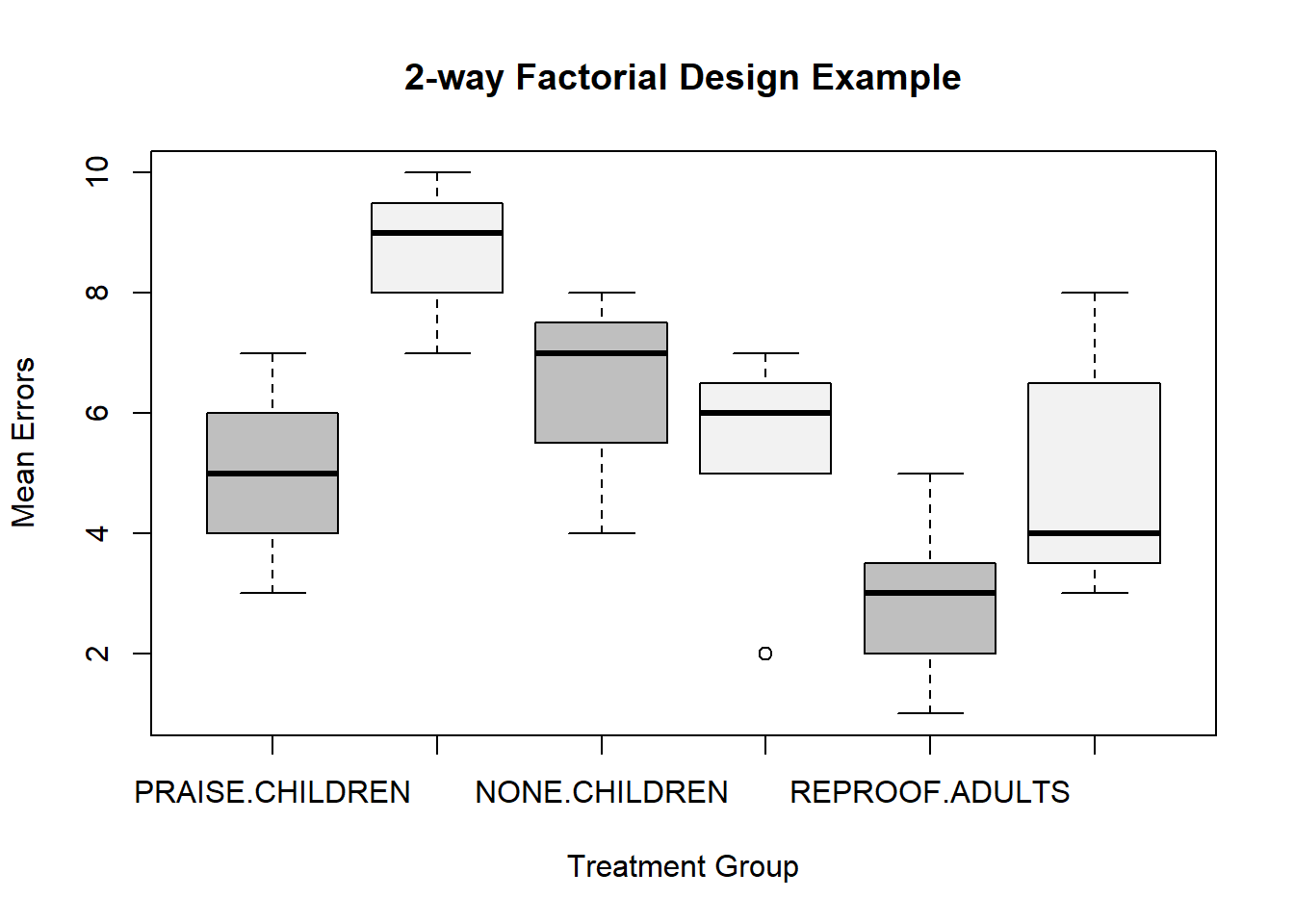### 2.2.5 Bar Graphs with error bars

The so-called “dynamite plot” is the standard graph for factorial design preseentation of means with standard errors. Initially, I used a function from the sciplot package called ‘bargraph.CI’. Its default implementation produces standard error bars (+/- 1 std error).

``````#require(sciplot)
#win.graph()
bargraph.CI(group,errors,group=age,lc=TRUE, uc=TRUE,legend=T,
cex.leg=1,bty="n",col=c("gray75","gray95"),ylim=c(0.01,9.9),
ylab="MEAN ERRORS",main="Base 2-Way Design Illustration")
box()
axis(4,labels=F)``````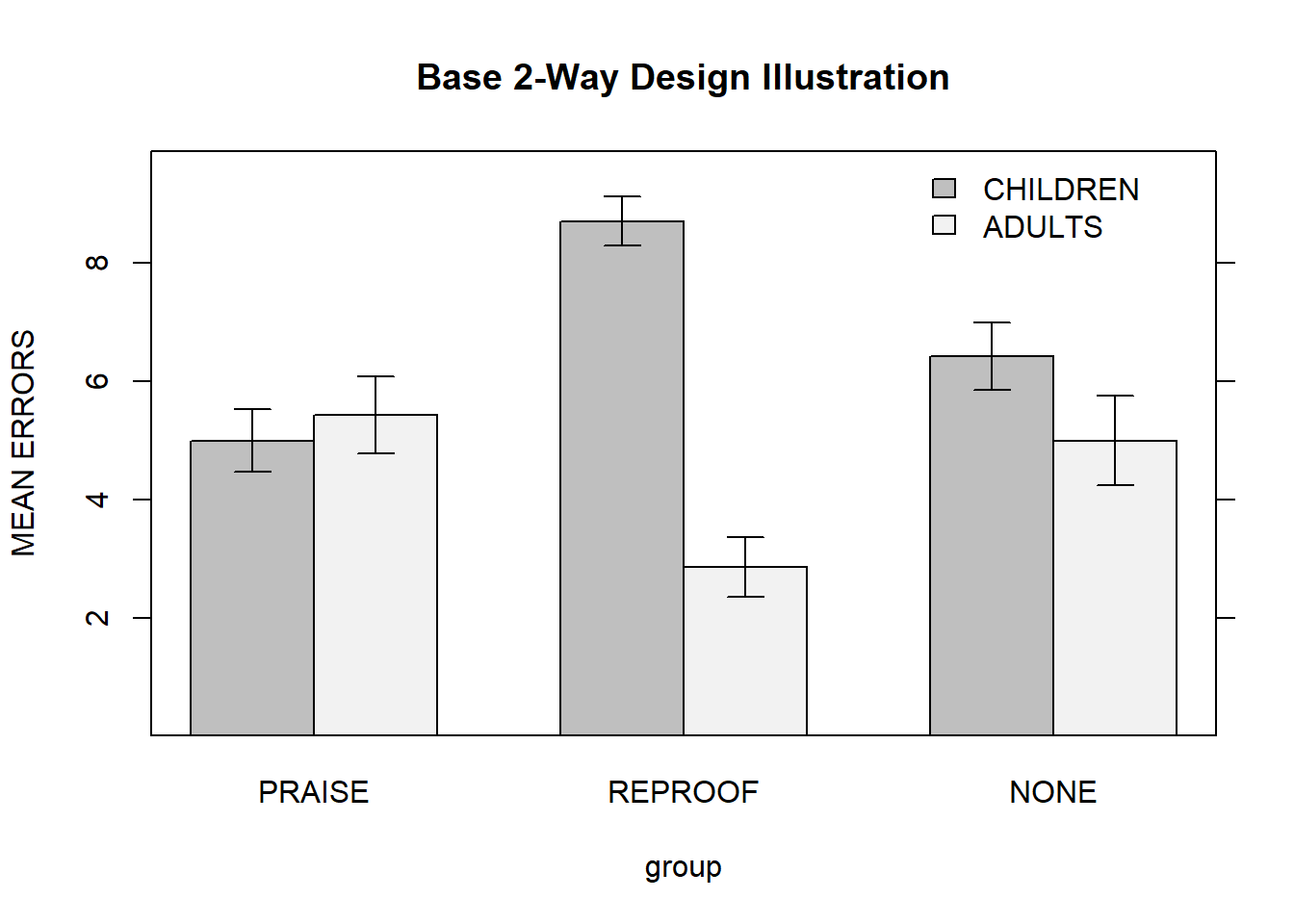## 2.3 Clustered bar charts with ggplot2

First, we need to summarize the data set to produce a new data frame that ggplot can used with ggplot2. This uses a function from the Rmisc package that extracts the means/SDs from the original data frame and produces a new data frame that has just means and std errors for the groups. The function returns a data frame that contains the means, standard deviations, std errors and CI’s for each of the groups. We might use any of the three indices of dispersion on our bar chart.

``````myData <- Rmisc::summarySE(data=prn.data, groupvars=c("group","age"), measurevar="errors" )
myData``````
``````##     group      age N   errors       sd        se       ci
## 1  PRAISE CHILDREN 7 5.000000 1.414214 0.5345225 1.307929
## 2  PRAISE   ADULTS 7 5.428571 1.718249 0.6494372 1.589116
## 3 REPROOF CHILDREN 7 8.714286 1.112697 0.4205600 1.029073
## 4 REPROOF   ADULTS 7 2.857143 1.345185 0.5084323 1.244089
## 5    NONE CHILDREN 7 6.428571 1.511858 0.5714286 1.398235
## 6    NONE   ADULTS 7 5.000000 2.000000 0.7559289 1.849691``````

Next I produce a relatively finished graph. Note that it would be easy to switch colors from my favored honeydew shades to a set of grays by changing the color names in the ‘scale_fill_manual’ ggplot argument.

``````#library(ggplot2)
#library(ggthemes)
# Default bar plot
p<- ggplot(myData, aes(x=group, y=errors, fill=age)) +
geom_bar(stat="identity", color="black",
position=position_dodge()) +
geom_errorbar(aes(ymin=errors-se, ymax=errors+se), width=.2,
position=position_dodge(.9))
#win.graph()
#print(p)
# a bit more finished bar plot
p2 <- p+labs(title="Errors by Treatment and Age +/- SEM", x="Treatment Group", y = "Mean Errors") +
theme_classic() +
theme(panel.grid.major.x = element_blank(),
panel.grid.major.y = element_blank(),
panel.grid.minor.x = element_blank(),
panel.grid.minor.y = element_blank(),
panel.background = element_blank(),
axis.line.y = element_line(colour="black", size=.7),
axis.line.x = element_line(colour="black", size=.7),
plot.title = element_text(hjust=.5)
) +
coord_cartesian(ylim=c(0, 9.25)) +  scale_y_continuous(expand = c(0,0)) +
theme(legend.justification=c(0,0), legend.position=c(0,.8)) +
scale_fill_manual(values=c('honeydew3','honeydew2'))``````
``````## Warning: The `size` argument of `element_line()` is deprecated as of ggplot2 3.4.0.
``````#win.graph()
print(p2)``````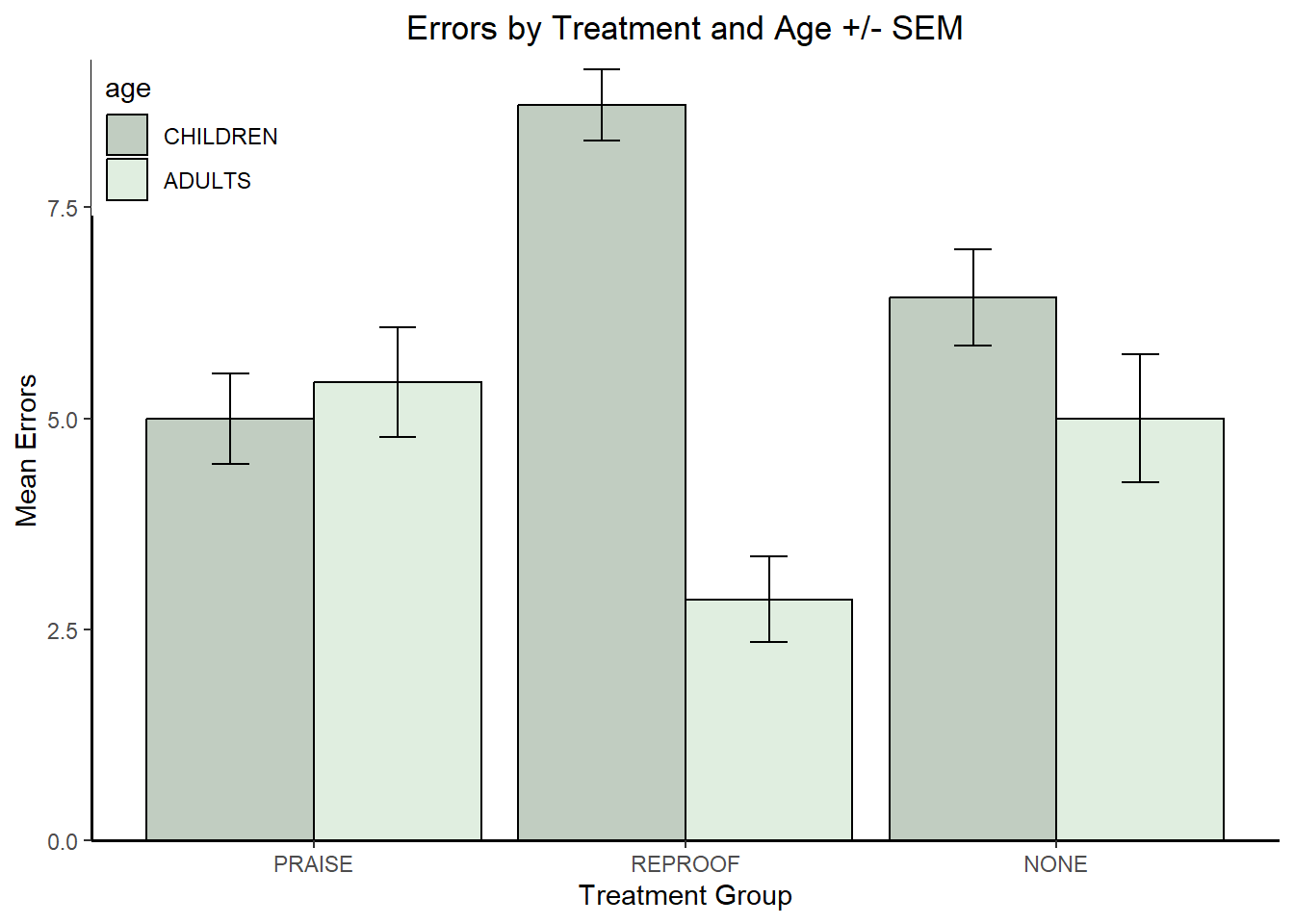# 3 Perform the basic/omnibus ANOVA

As we saw with the 1way design, both ‘aov’ and ‘lm’ can be employed to assess the 2way design. Both approaches are executed here.

In this and following sections where we can name the data frame in an argment in the `aov` or `lm` function, we don’t need the data frame to be attached. It is detached here:

``detach(prn.data)``

## 3.1 Using `aov` and `lm` for the omnibus 2-way factorial model

For factorial designs, the model specification can proceed one of two ways. A full model that contains both interactions and main effects could be specified as:

`errors ~ group + age + group:age`, where the colon notation specifies an interaction term.

But a more efficient way of specifying the model is to use

`errors ~ group*age`, where the asterisk term results in production of both the higher order interaction term and the lower order main effects.

Here, using ‘aov’, we see that the ‘summary’ and ‘anova’ functions give similar ANOVA summary tables. Notice that the interaction term are always written using the colon notation.

``````# We can do the 2 way ANOVA with AOV
fit.1aov <- aov(errors~ group*age, data=prn.data)
summary(fit.1aov)``````
``````##             Df Sum Sq Mean Sq F value   Pr(>F)
## group        2   2.71    1.36    0.57    0.571
## age          1  54.86   54.86   23.04 2.76e-05 ***
## group:age    2  73.00   36.50   15.33 1.53e-05 ***
## Residuals   36  85.71    2.38
## ---
## Signif. codes:  0 '***' 0.001 '**' 0.01 '*' 0.05 '.' 0.1 ' ' 1``````
``anova(fit.1aov)``
``````## Analysis of Variance Table
##
## Response: errors
##           Df Sum Sq Mean Sq F value    Pr(>F)
## group      2  2.714   1.357    0.57    0.5705
## age        1 54.857  54.857   23.04 2.764e-05 ***
## group:age  2 73.000  36.500   15.33 1.527e-05 ***
## Residuals 36 85.714   2.381
## ---
## Signif. codes:  0 '***' 0.001 '**' 0.01 '*' 0.05 '.' 0.1 ' ' 1``````
``#Anova(fit.1aov, type=3)``

When we use ‘lm’, the ‘summary’ and ‘anova’ functions give different tables. `Summary` produces a listing of all individual regression coefficients (“estimates”) and their std errors and t-tests. This prompts a question of what the coding scheme is that R has used by default and we consider that in the next section. Use of `anova` produces the expected ANOVA summary table of SS/MS/DF/F’s/p’s with multiple df sources listed rather than all single df terms.

``````# Or, we can do the 2 way anova with the lm function (same outcome)
fit.1lm <- lm(errors~group*age, data=prn.data)
summary(fit.1lm)``````
``````##
## Call:
## lm(formula = errors ~ group * age, data = prn.data)
##
## Residuals:
##     Min      1Q  Median      3Q     Max
## -3.4286 -1.0000  0.1429  1.1071  3.0000
##
## Coefficients:
##                        Estimate Std. Error t value Pr(>|t|)
## (Intercept)              5.0000     0.5832   8.573 3.21e-10 ***
## groupREPROOF             3.7143     0.8248   4.503 6.78e-05 ***
## groupNONE                1.4286     0.8248   1.732   0.0918 .
## ageADULTS                0.4286     0.8248   0.520   0.6065
## groupREPROOF:ageADULTS  -6.2857     1.1664  -5.389 4.56e-06 ***
## groupNONE:ageADULTS     -1.8571     1.1664  -1.592   0.1201
## ---
## Signif. codes:  0 '***' 0.001 '**' 0.01 '*' 0.05 '.' 0.1 ' ' 1
##
## Residual standard error: 1.543 on 36 degrees of freedom
## Multiple R-squared:  0.6037, Adjusted R-squared:  0.5487
## F-statistic: 10.97 on 5 and 36 DF,  p-value: 1.823e-06``````
``anova(fit.1lm)``
``````## Analysis of Variance Table
##
## Response: errors
##           Df Sum Sq Mean Sq F value    Pr(>F)
## group      2  2.714   1.357    0.57    0.5705
## age        1 54.857  54.857   23.04 2.764e-05 ***
## group:age  2 73.000  36.500   15.33 1.527e-05 ***
## Residuals 36 85.714   2.381
## ---
## Signif. codes:  0 '***' 0.001 '**' 0.01 '*' 0.05 '.' 0.1 ' ' 1``````

## 3.2 Coding scheme used for default analyses

The ‘lm’ summary object above included regression coefficient for five coding vectors. What are the “contrasts” employed as the default?

``contrasts(prn.data\$group)``
``````##         REPROOF NONE
## PRAISE        0    0
## REPROOF       1    0
## NONE          0    1``````
``contrasts(prn.data\$age)``
``````##          ADULTS
## CHILDREN      0

This reveals that R creates default coding vectors for each IV. But the age IV only requires one since it is just a two level variable. The pattern of coefficients for the treatment group IV clearly reveal that R is using dummy coding by default (it may be called indicator coding in some help docs and could have been specified with `contr.treatment` as we saw with the 1-way design tutorial). The first group (praise) is the reference category, by default (and could be changed if desired). The regression coefficients in the output of the summary(fit.1lm) code above should now be interpretable since we know the coding scheme. It is worthwhile to contemplate what the product vectors were that produced the interaction vectors.

## 3.3 Type I, II, and III SS

With our equal N design, these three types of SS are equivalent. If we had unequal N, we might want to employ Type 2 or 3 SS. We can use the ‘Anova’ function from car to obtain these. However, ‘Anova’ will not provide proper computations if we continue to use dummy coding for the model. So, we can change this to “zero sum” contrasts by choosing effect coding. Later in this document we will use orthogonal contrast coding which will also suffice, and provides the analyst with complete control over contrast specification.

``````#options(conrasts=c("contr.sum","contr.poly"))
contrasts(prn.data\$group)=contr.sum
contrasts(prn.data\$age)=contr.sum
contrasts(prn.data\$group)``````
``````##         [,1] [,2]
## PRAISE     1    0
## REPROOF    0    1
## NONE      -1   -1``````
``contrasts(prn.data\$age)``
``````##          [,1]
## CHILDREN    1

Now, refitting the base `aov` model uses these newly specified contrasts. Note that coding vector choice does not affect the omnibus F test outcomes. The same SS/MS/F/p values emerge

``````fit.1aovb <- aov(errors~group*age, data=prn.data)
#anova(fit.1aovb)
Anova(fit.1aovb, type=3)``````
``````## Anova Table (Type III tests)
##
## Response: errors
##              Sum Sq Df F value    Pr(>F)
## (Intercept) 1303.71  1  547.56 < 2.2e-16 ***
## group          2.71  2    0.57    0.5705
## age           54.86  1   23.04 2.764e-05 ***
## group:age     73.00  2   15.33 1.527e-05 ***
## Residuals     85.71 36
## ---
## Signif. codes:  0 '***' 0.001 '**' 0.01 '*' 0.05 '.' 0.1 ' ' 1``````

However, if we examine the output with `summary.lm` to see the individual regression coefficients and tests, these will be different than those seen above. With “effect” coding the intercept has a value equal to the grand mean of the DV, rather than the mean of a reference category as seen above with indicator coding. And the regression coefficients have values equivalent to the deviation of individual groups from the grand mean. The hypotheses about these individuals group deviations from the grand mean my not be of interest to the researcher, so the illustration of “effect” coding here is provided simply as an alternative to dummy coding for production of the omnibus F tests of main effects and interactions. Work found in a later section of this document, with orthogonal/analytical contrasts is a preferred strategy.

``summary.lm(fit.1aovb)``
``````##
## Call:
## aov(formula = errors ~ group * age, data = prn.data)
##
## Residuals:
##     Min      1Q  Median      3Q     Max
## -3.4286 -1.0000  0.1429  1.1071  3.0000
##
## Coefficients:
##             Estimate Std. Error t value Pr(>|t|)
## (Intercept)   5.5714     0.2381  23.400  < 2e-16 ***
## group1       -0.3571     0.3367  -1.061 0.295909
## group2        0.2143     0.3367   0.636 0.528544
## age1          1.1429     0.2381   4.800 2.76e-05 ***
## group1:age1  -1.3571     0.3367  -4.031 0.000276 ***
## group2:age1   1.7857     0.3367   5.303 5.93e-06 ***
## ---
## Signif. codes:  0 '***' 0.001 '**' 0.01 '*' 0.05 '.' 0.1 ' ' 1
##
## Residual standard error: 1.543 on 36 degrees of freedom
## Multiple R-squared:  0.6037, Adjusted R-squared:  0.5487
## F-statistic: 10.97 on 5 and 36 DF,  p-value: 1.823e-06``````

## 3.4 Effect sizes for the omnibus analysis

The sjstats package has a function that computes several useful effect size statistics and it works well with two-factor designs. The ’anova_stats function does the analysis and also provides effect sizes. Note that this default approach will produce effect sizes based on Type I SS. If Type III are desired, see the section two code chunks below.

``````# the gt function permits nicer formatting of the table
anova_stats(fit.1aov)``````
``````## term      | df |  sumsq | meansq | statistic | p.value | etasq | partial.etasq | omegasq | partial.omegasq | epsilonsq | cohens.f | power
## -----------------------------------------------------------------------------------------------------------------------------------------
## group     |  2 |  2.714 |  1.357 |     0.570 |   0.571 | 0.013 |         0.031 |  -0.009 |          -0.021 |    -0.009 |    0.178 | 0.145
## age       |  1 | 54.857 | 54.857 |    23.040 |  < .001 | 0.254 |         0.390 |   0.240 |           0.344 |     0.243 |    0.800 | 0.998
## group:age |  2 | 73.000 | 36.500 |    15.330 |  < .001 | 0.338 |         0.460 |   0.312 |           0.406 |     0.315 |    0.923 | 0.999
## Residuals | 36 | 85.714 |  2.381 |           |         |       |               |         |                 |           |          |``````
``#gt(anova_stats(fit.1aov))``

Or, we could specify an indidual set of effect sizes which would then be accompanied by confidence intervals. Note that since effect sizes cannot be negative, the smallest lower boundary for a CI would be zero. This happens whenever a term is NS at an alpha level equivalent to the CI level (e.g., .05 alpha and .95 CI level). This outcome occurred for the main effect of group in this data set.

``effectsize::eta_squared(fit.1aov, partial=TRUE, ci=.95, alternative="two")``
``````## # Effect Size for ANOVA (Type I)
##
## Parameter | Eta2 (partial) |       95% CI
## -----------------------------------------
## group     |           0.03 | [0.00, 0.17]
## age       |           0.39 | [0.15, 0.58]
## group:age |           0.46 | [0.20, 0.63]``````

For future reference, when designs have unequal N, the following approach can compute these effect sizes using Type III SS, if that is desirable. ‘anova_stats’ can work with a model object produced by the ‘Anova’ function (upper case first letter A) from car, which permits Type II or III specification. Notice that we had to use the model object where the default coding was changed from dummy to zero-sum or effect coding.

``````#options(conrasts=c("contr.sum","contr.poly"))
anova_stats(Anova(fit.1aovb, type = "III"))``````
``````## term      |  sumsq | meansq | df | statistic | p.value | etasq | partial.etasq | omegasq | partial.omegasq | epsilonsq | cohens.f | power
## -----------------------------------------------------------------------------------------------------------------------------------------
## group     |  2.714 |  1.357 |  2 |     0.570 |   0.571 | 0.013 |         0.031 |  -0.009 |          -0.021 |    -0.009 |    0.178 | 0.145
## age       | 54.857 | 54.857 |  1 |    23.040 |  < .001 | 0.254 |         0.390 |   0.240 |           0.344 |     0.243 |    0.800 | 0.998
## group:age | 73.000 | 36.500 |  2 |    15.330 |  < .001 | 0.338 |         0.460 |   0.312 |           0.406 |     0.315 |    0.923 | 0.999
## Residuals | 85.714 |  2.381 | 36 |           |         |       |               |         |                 |           |          |``````

## 3.5 “Manual” computation of effect sizes for verification and illustration.

To illustrate a way of using information in the fit object we can “manually” extract the relevant SS from the AOV model fit object. This permits computation of several effect size statistics “by hand”. Here I compute the partial eta squareds. Notice that the results matche the values produced by the ‘anova_stats’ function.

``````fit.1SS <- anova(fit.1aov)
SStreat   <- fit.1SS["group", "Sum Sq"]
SSage   <- fit.1SS["age", "Sum Sq"]
SSInt   <- fit.1SS["group:age", "Sum Sq"]
SSErr   <- fit.1SS["Residuals", "Sum Sq"]
pEtaSq.treat <- SStreat / (SStreat + SSErr)
pEtaSq.age <- SSage / (SSage + SSErr)
pEtaSq.int <- SSInt / (SSInt + SSErr)
pEtaSq.treat``````
``##  0.03069467``
``pEtaSq.age``
``##  0.3902439``
``pEtaSq.int``
``##  0.459946``

## 3.6 Evaluate Assumptions of normality and homogeneity of variance

Next we produce the diagnostic plots for linear models and test normality. The `plot` function works on an `aov` object in the same way it does for `lm` objects. I have produced the two most important plots by extraction with the `which` argument. Even with the small sample sizes per group, these plots give some reassurance that any violation of homoscedasticity (homogeneity of variance) and normality assumptions is small for this data set.

``````#win.graph()
plot(fit.1aov,which=1)``````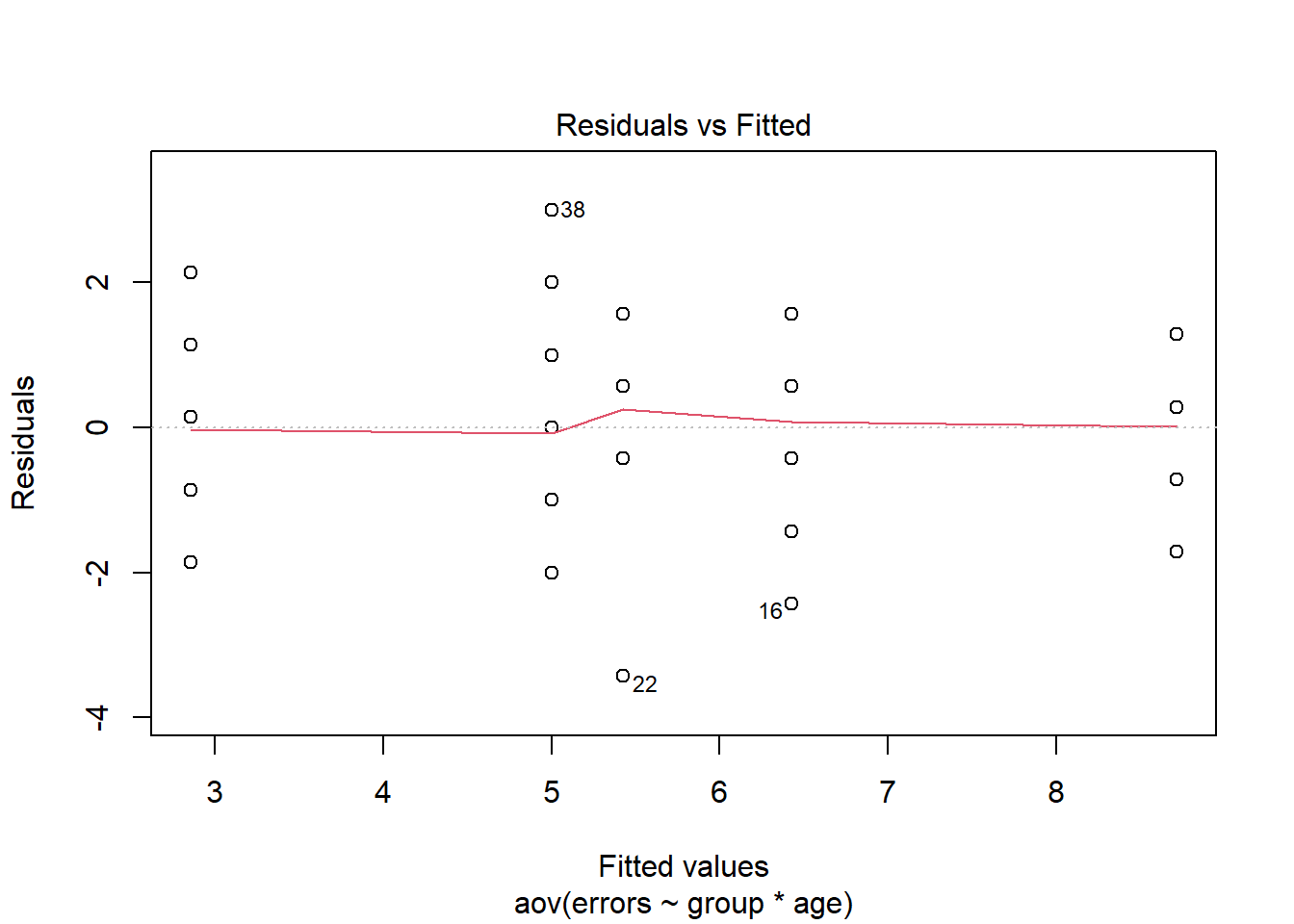``````#win.graph()
plot(fit.1aov,which=2)``````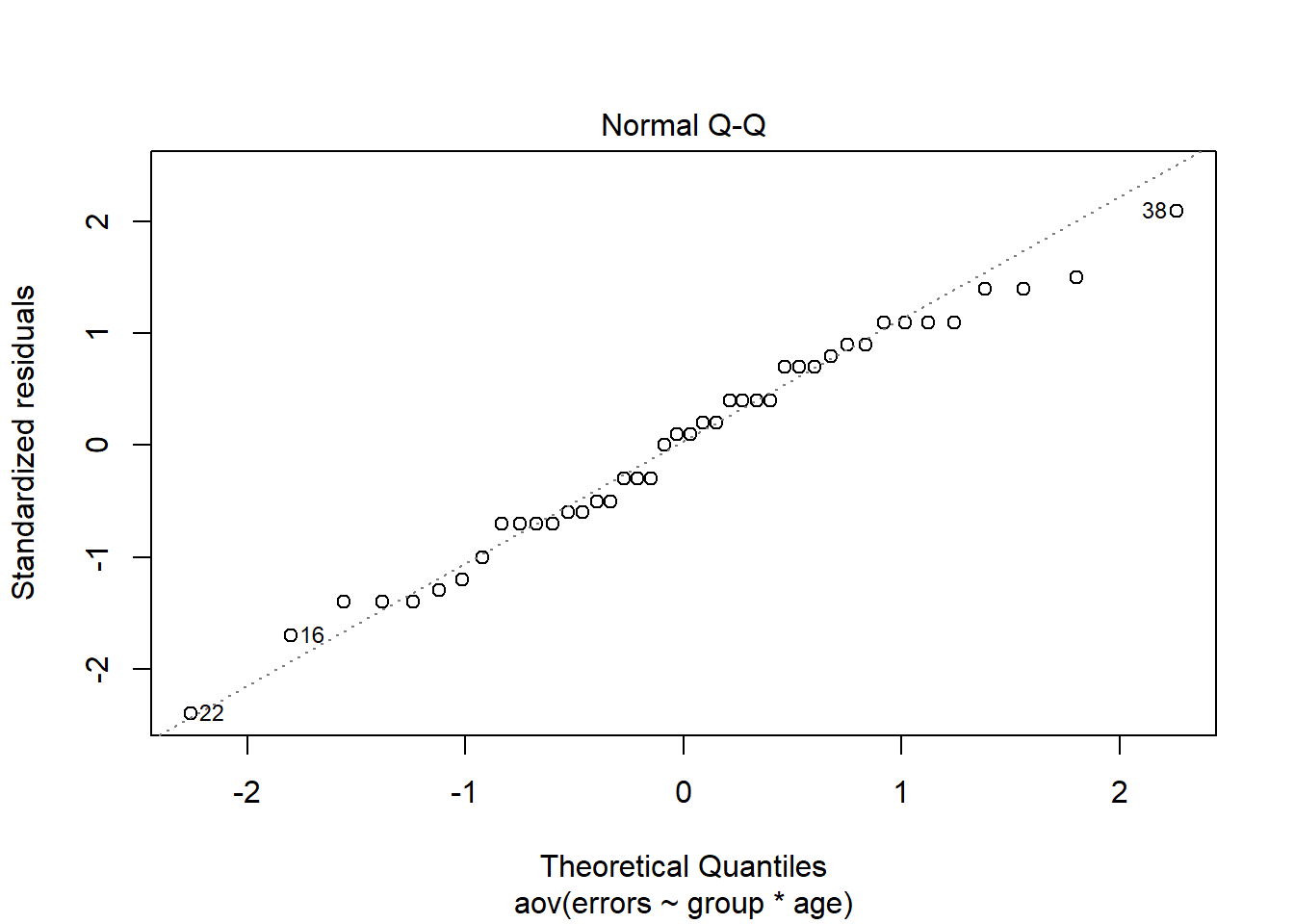``````#win.graph()
#plot(fit.1aov,which=3)
#win.graph()
#plot(fit.1aov,which=5)``````

Several tests of the assumption that residuals are normal are also available (review the larger set of possibilities in the 1-way tutorial document). Here, I used the Anderson-Darling test and provide commented code for the Shapiro-Wilk test.

``````# Perform the Shapiro-Wilk test for normality on the residuals
#shapiro.test(residuals(fit.1aov))
# perform the Anderson-Darling normality test on the residuals
``````##
##  Anderson-Darling normality test
##
## data:  residuals(fit.1aov)
## A = 0.31887, p-value = 0.5231``````

## 3.7 Test Homogeneity of Variance / Homoscedasticity

For illustration, I’ve done both the mean-centered and median=centered Levene Tests for Homogeneity of Variance (using the function from the car package). The median-centered version is preferred.

``````#     first use the mean-centering approach and recall that this
#     is what SPSS uses in one-way and GLM
car::leveneTest(errors~group*age,center=mean,data=prn.data)``````
``````## Levene's Test for Homogeneity of Variance (center = mean)
##       Df F value Pr(>F)
## group  5  0.8957 0.4944
##       36``````
``````#     then with median centering to match levene.test from car, and
#     to provide the Brown-Forsythe method that was the modification of Levene
#     to be more robust against non-normality
car::leveneTest(errors~group*age,center=median,data=prn.data)``````
``````## Levene's Test for Homogeneity of Variance (center = median)
##       Df F value Pr(>F)
## group  5    0.35 0.8789
##       36``````

# 4 Orthogonal Analytical Contrasts

We will want to implement orthogonal contrast coding for the treatment group factor. Age, with only two levels is already a 1df contrast. Nonetheless, I specified the contrast for age directly as a placeholder to remind us that we need to specify contrasts for each factor separately (for larger designs when both factors have more than two levels)

``````# Let's create contrasts of interest to us.
# The same orthogonal set we used in our SPSS work with this data set.
contrasts.group <- matrix(c(-1,2,-1,1,0,-1),ncol=2)
contrasts(prn.data\$group) <- contrasts.group
contrasts(prn.data\$group)``````
``````##         [,1] [,2]
## PRAISE    -1    1
## REPROOF    2    0
## NONE      -1   -1``````

For the sake of completeness, we will specify that age also uses a sum to zero analytical contrast. But since it is only a two-level factor, this contrast is equivalent to effect coding for which the variable is already set by the code in a previous section.

``````contrasts.age <- matrix(c(1,-1),ncol=1)
contrasts(prn.data\$age) <- contrasts.age
contrasts(prn.data\$age)``````
``````##          [,1]
## CHILDREN    1

## 4.1 Obtain tests of these contrasts with `aov` and `lm` modeling

We can apply these contrasts to the AOV and/or lm models from above, simply by rerunning them. The ‘split’ argument is used in a manner similar to what we did in 1-way designs. When ‘split’ sees the factor name “group” it knows to look for two contrasts (and we have named them ac1 and ac2). This results in a decomposition of both the main effect of group, and the interaction. Once again, ‘summary’ does different things on the ‘aov’ and ‘lm’ objects.

``````fit.2aov <- aov(errors~group*age, data=prn.data)
fit.2lm <- lm(errors~group*age, data=prn.data)
summary(fit.2aov,  split=list(group=list(group.ac1=1, group.ac2=2)))``````
``````##                        Df Sum Sq Mean Sq F value   Pr(>F)
## group                   2   2.71    1.36   0.570    0.571
##   group: group.ac1      1   0.96    0.96   0.405    0.529
##   group: group.ac2      1   1.75    1.75   0.735    0.397
## age                     1  54.86   54.86  23.040 2.76e-05 ***
## group:age               2  73.00   36.50  15.330 1.53e-05 ***
##   group:age: group.ac1  1  66.96   66.96  28.125 5.93e-06 ***
##   group:age: group.ac2  1   6.04    6.04   2.535    0.120
## Residuals              36  85.71    2.38
## ---
## Signif. codes:  0 '***' 0.001 '**' 0.01 '*' 0.05 '.' 0.1 ' ' 1``````
``summary(fit.2lm,  split=list(group=list(group.ac1=1, group.ac2=2)))``
``````##
## Call:
## lm(formula = errors ~ group * age, data = prn.data)
##
## Residuals:
##     Min      1Q  Median      3Q     Max
## -3.4286 -1.0000  0.1429  1.1071  3.0000
##
## Coefficients:
##             Estimate Std. Error t value Pr(>|t|)
## (Intercept)   5.5714     0.2381  23.400  < 2e-16 ***
## group1        0.1071     0.1684   0.636    0.529
## group2       -0.2500     0.2916  -0.857    0.397
## age1          1.1429     0.2381   4.800 2.76e-05 ***
## group1:age1   0.8929     0.1684   5.303 5.93e-06 ***
## group2:age1  -0.4643     0.2916  -1.592    0.120
## ---
## Signif. codes:  0 '***' 0.001 '**' 0.01 '*' 0.05 '.' 0.1 ' ' 1
##
## Residual standard error: 1.543 on 36 degrees of freedom
## Multiple R-squared:  0.6037, Adjusted R-squared:  0.5487
## F-statistic: 10.97 on 5 and 36 DF,  p-value: 1.823e-06``````
``#summary.lm(fit.2aov) #see below``

The ANOVA summary Tables from ‘anova’ and ‘Anova” do not partition the 2-df sources into single df effects. Instead, then return only the Type I or Type II SS omnibus ANOVA summary tables. This gives four different tables containing the identical result. And, since ’summary’ on the aov object above gave the omnibus sources plus contrasts, it would be the preferred approach (unless Type III SS were needed). For tests of Type III SS of contrasts and their effect sizes, we use the emmeans and phia approaches found in later sections in this document, or the immediately succeeding code chunk here that uses `summary.lm`.

``anova(fit.2aov)``
``````## Analysis of Variance Table
##
## Response: errors
##           Df Sum Sq Mean Sq F value    Pr(>F)
## group      2  2.714   1.357    0.57    0.5705
## age        1 54.857  54.857   23.04 2.764e-05 ***
## group:age  2 73.000  36.500   15.33 1.527e-05 ***
## Residuals 36 85.714   2.381
## ---
## Signif. codes:  0 '***' 0.001 '**' 0.01 '*' 0.05 '.' 0.1 ' ' 1``````
``anova(fit.2lm)``
``````## Analysis of Variance Table
##
## Response: errors
##           Df Sum Sq Mean Sq F value    Pr(>F)
## group      2  2.714   1.357    0.57    0.5705
## age        1 54.857  54.857   23.04 2.764e-05 ***
## group:age  2 73.000  36.500   15.33 1.527e-05 ***
## Residuals 36 85.714   2.381
## ---
## Signif. codes:  0 '***' 0.001 '**' 0.01 '*' 0.05 '.' 0.1 ' ' 1``````
``````# now produce Type III SS with the Anova function
# note that the Anova function in car will not produce the expected results
# on AOV or lm model objects when the "contrasts" employed are dummy or effect coding.
# since the default coding is dummy coding, as seen above, Anova should only be used
# for type 3 SS after contrasts are changed to zero-summing contrasts, as
# we have done here, implementing the orthogonal contrasts for each variable.
require(car)
Anova(fit.2aov,type=3)``````
``````## Anova Table (Type III tests)
##
## Response: errors
##              Sum Sq Df F value    Pr(>F)
## (Intercept) 1303.71  1  547.56 < 2.2e-16 ***
## group          2.71  2    0.57    0.5705
## age           54.86  1   23.04 2.764e-05 ***
## group:age     73.00  2   15.33 1.527e-05 ***
## Residuals     85.71 36
## ---
## Signif. codes:  0 '***' 0.001 '**' 0.01 '*' 0.05 '.' 0.1 ' ' 1``````
``Anova(fit.2lm, type=3)``
``````## Anova Table (Type III tests)
##
## Response: errors
##              Sum Sq Df F value    Pr(>F)
## (Intercept) 1303.71  1  547.56 < 2.2e-16 ***
## group          2.71  2    0.57    0.5705
## age           54.86  1   23.04 2.764e-05 ***
## group:age     73.00  2   15.33 1.527e-05 ***
## Residuals     85.71 36
## ---
## Signif. codes:  0 '***' 0.001 '**' 0.01 '*' 0.05 '.' 0.1 ' ' 1``````

## 4.2 Using `summary.lm` to obtain tests of single df contrasts

We have seen that using the “split” argument in `summary` on an `aov` object is an efficient way of obtaining SS decompositions and F tests of contrasts. However, these will be Type I SS in unbalanced designs. We had seen in the 1-way tutorial that efficient way of obtaining inferences on single df contrasts in an `aov` object is to use the `summary.lm` function. This approach produces t-tests rather than F tests, but they are equivalent since the squares of the t’s are the F values. But in cases of unequal N, this equivalence is for Type III tests - an outcome that is desirable in most experimental circumstances with factorial designs. The only downside to using this approach is that it does not produce the individual SS for the contrasts, so are not available for things like manual computation of effect sizes.

``summary.lm(fit.2aov)``
``````##
## Call:
## aov(formula = errors ~ group * age, data = prn.data)
##
## Residuals:
##     Min      1Q  Median      3Q     Max
## -3.4286 -1.0000  0.1429  1.1071  3.0000
##
## Coefficients:
##             Estimate Std. Error t value Pr(>|t|)
## (Intercept)   5.5714     0.2381  23.400  < 2e-16 ***
## group1        0.1071     0.1684   0.636    0.529
## group2       -0.2500     0.2916  -0.857    0.397
## age1          1.1429     0.2381   4.800 2.76e-05 ***
## group1:age1   0.8929     0.1684   5.303 5.93e-06 ***
## group2:age1  -0.4643     0.2916  -1.592    0.120
## ---
## Signif. codes:  0 '***' 0.001 '**' 0.01 '*' 0.05 '.' 0.1 ' ' 1
##
## Residual standard error: 1.543 on 36 degrees of freedom
## Multiple R-squared:  0.6037, Adjusted R-squared:  0.5487
## F-statistic: 10.97 on 5 and 36 DF,  p-value: 1.823e-06``````

## 4.3 Manually Compute partial eta squareds on Type I contrast SS

``````# compute partial eta squareds on Type III SS (same as Type I here since
# we have equal N)
fit.2SS <- Anova(fit.2aov)
SStreat2   <- fit.2SS["group",       "Sum Sq"]
SSage2   <- fit.2SS["age",       "Sum Sq"]
SSInt2   <- fit.2SS["group:age",   "Sum Sq"]
SSErr2   <- fit.2SS["Residuals", "Sum Sq"]
pEtaSq2.treat <- SStreat2 / (SStreat2 + SSErr2)
pEtaSq2.age <- SSage2 / (SSage2 + SSErr2)
pEtaSq2.int <- SSInt2 / (SSInt2 + SSErr2)
pEtaSq2.treat``````
``##  0.03069467``
``pEtaSq2.age``
``##  0.3902439``
``pEtaSq2.int``
``##  0.459946``
``````# Note: To obtain partial eta squareds on the single df contrasts,
# save the SS partition table from the summary function and manually extract
# following ths strategy (the summary function is the same one from above)
SS.contrastmodel2 <- summary(fit.2aov,
split=list(group=list(group.ac1=1,
group.ac2=2)))
SS.contrastmodel2 # look at the SS table to identify where the relevant SS are``````
``````##                        Df Sum Sq Mean Sq F value   Pr(>F)
## group                   2   2.71    1.36   0.570    0.571
##   group: group.ac1      1   0.96    0.96   0.405    0.529
##   group: group.ac2      1   1.75    1.75   0.735    0.397
## age                     1  54.86   54.86  23.040 2.76e-05 ***
## group:age               2  73.00   36.50  15.330 1.53e-05 ***
##   group:age: group.ac1  1  66.96   66.96  28.125 5.93e-06 ***
##   group:age: group.ac2  1   6.04    6.04   2.535    0.120
## Residuals              36  85.71    2.38
## ---
## Signif. codes:  0 '***' 0.001 '**' 0.01 '*' 0.05 '.' 0.1 ' ' 1``````
``````# for example, the SS from the first interaction contrast.
SS.intcontr1 <- SS.contrastmodel2[]["Sum Sq"][6,]  # relevant SS from sixth row of object
SS.intcontr1``````
``````## group.ac1
##  66.96429``````
``````# now SS resid
SS.error <- SS.contrastmodel2[]["Residuals", "Sum Sq"]
SS.error``````
``````##
## 85.71429``````
``````# now partial eta squared for this first interaction contrast:
pEtaSq.intcontr1 <- SS.intcontr1/(SS.intcontr1 + SS.error)
pEtaSq.intcontr1``````
``````## group.ac1
## 0.4385965``````

I am not aware of a built-in way of obtaining SS for individual Type III SS so that eta and partial eta squareds can be computed manually. This is revisited in a later section.

# 5 Take a unique approach to plotting the 2 way model with granova

NOTE that ‘granova.2w’ produces an interactive 3D plot that will not render with R markdown, but I’ve included a screen capture below this code chunk’s output. Running this code independently from the markdown document will produce the plot in a separate graphics window.

``````# require(granova)
# granova requires the first variable in the data frame to be the dv, so.....
prn.data2 <- as.data.frame(cbind(prn.data\$errors,prn.data\$group,prn.data\$age))
colnames(prn.data2) <- c("errors","group","age")
#prn.data2
granova.2w(data=prn.data2,formula=errors~group*age)``````
``## Loading required package: rgl``
``## Loading required package: tcltk``
``## Loading required package: mgcv``
``## Loading required package: nlme``
``````##
## Attaching package: 'nlme'``````
``````## The following object is masked from 'package:lme4':
##
##     lmList``````
``## This is mgcv 1.8-41. For overview type 'help("mgcv-package")'.``
``````## \$group.effects
##      1      3      2
## -0.357  0.143  0.214
##
## \$age.effects
##     2     1
## -1.14  1.14
##
## \$CellCounts.Reordered
##      age
## group 2 1
##     1 7 7
##     3 7 7
##     2 7 7
##
## \$CellMeans.Reordered
##      age
## group    2    1
##     1 5.43 5.00
##     3 5.00 6.43
##     2 2.86 8.71
##
## \$aov.summary
##             Df Sum Sq Mean Sq F value   Pr(>F)
## group        2   2.71    1.36    0.57    0.571
## age          1  54.86   54.86   23.04 2.76e-05 ***
## group:age    2  73.00   36.50   15.33 1.53e-05 ***
## Residuals   36  85.71    2.38
## ---
## Signif. codes:  0 '***' 0.001 '**' 0.01 '*' 0.05 '.' 0.1 ' ' 1``````

The plot fits an additive model plane for a two-IV model so that the deviation of group means from the expected position based on an additive model can help visualize any interaction.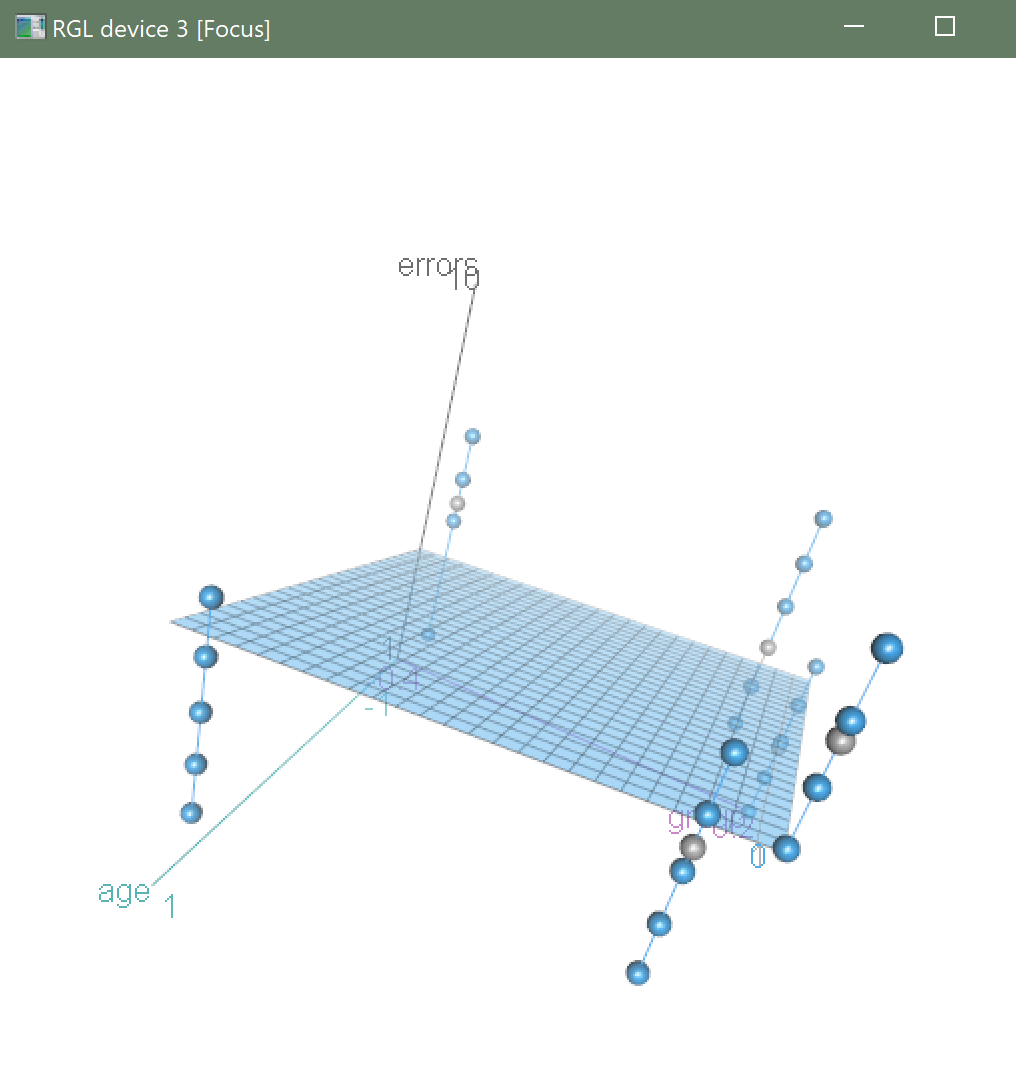granova.2W 3D plot

The reader is encouraged to use the Moderator models shiny app that is provided for class purposes in the bcdstats package to see more detailed implementations of surface fitting in additive and interactive models.

# 6 Obtain Simple Main Effects and all Contrasts, using the phia package.

The phia package provides strong tools for exploring interactions. It will produce tests of contrasts and simple main effects, including main effect contrasts, interaction contrasts, simple main effects, and simple main effect contrasts. It appears to work well here with this balanced design (equal N), but extension to other kinds of simple effects, and to repeated measures deisgns may be challenging.

It appears to produce tests of Type III SS which usually is recommended for experimental work, but we cover that topic later in the course as we consider “unbalanced” designs” with unequal sample sizes.

Initially, we can repeat the omnibus anova, making sure that the proper contrasts are in place for the group factor. Even though age is only a 2-level factor, the contrast is switched to what would either be effect coding or contrast coding since initially R would have it as dummy coding.

``````# Let's rework the whole analysis from the beginning.
# Note that I changed the first contrast to use fractions rather than the original
# -1,2,-1 from above.  This will help match up SME psi values with SME contrast psi values
contrasts.group <- matrix(c(-.5,1,-.5,1,0,-1),ncol=2)
contrasts(prn.data\$group) <- contrasts.group
contrasts(prn.data\$group)``````
``````##         [,1] [,2]
## PRAISE  -0.5    1
## REPROOF  1.0    0
## NONE    -0.5   -1``````
``````contrasts.age <- matrix(c(1,-1),ncol=1)
contrasts(prn.data\$age) <- contrasts.age
contrasts(prn.data\$age)``````
``````##          [,1]
## CHILDREN    1
``````# repeat the AOV with our othorgonal contrasts to make sure the correct fit model is
# employed.  Same as analyses above, to make sure fit objects match.
fit.2aov <- aov(errors~group*age, data=prn.data)
fit.2lm <- lm(errors~group*age, data=prn.data)``````

With the model objects in place we can view them. Both the ‘aov’ and ‘lm’ results are requested using ‘summary’ and the ‘split’ argument. This duplicates what was done earlier in this document for comparison to the results with the phia package to follow.

``````# this first summary function yields Type I SS if data set is unequal N
summary(fit.2aov,  split=list(group=list(group.ac1=1, group.ac2=2)))``````
``````##                        Df Sum Sq Mean Sq F value   Pr(>F)
## group                   2   2.71    1.36   0.570    0.571
##   group: group.ac1      1   0.96    0.96   0.405    0.529
##   group: group.ac2      1   1.75    1.75   0.735    0.397
## age                     1  54.86   54.86  23.040 2.76e-05 ***
## group:age               2  73.00   36.50  15.330 1.53e-05 ***
##   group:age: group.ac1  1  66.96   66.96  28.125 5.93e-06 ***
##   group:age: group.ac2  1   6.04    6.04   2.535    0.120
## Residuals              36  85.71    2.38
## ---
## Signif. codes:  0 '***' 0.001 '**' 0.01 '*' 0.05 '.' 0.1 ' ' 1``````
``````# this second summary function yields Type III tests if data set is unequal N
summary(fit.2lm)``````
``````##
## Call:
## lm(formula = errors ~ group * age, data = prn.data)
##
## Residuals:
##     Min      1Q  Median      3Q     Max
## -3.4286 -1.0000  0.1429  1.1071  3.0000
##
## Coefficients:
##             Estimate Std. Error t value Pr(>|t|)
## (Intercept)   5.5714     0.2381  23.400  < 2e-16 ***
## group1        0.2143     0.3367   0.636    0.529
## group2       -0.2500     0.2916  -0.857    0.397
## age1          1.1429     0.2381   4.800 2.76e-05 ***
## group1:age1   1.7857     0.3367   5.303 5.93e-06 ***
## group2:age1  -0.4643     0.2916  -1.592    0.120
## ---
## Signif. codes:  0 '***' 0.001 '**' 0.01 '*' 0.05 '.' 0.1 ' ' 1
##
## Residual standard error: 1.543 on 36 degrees of freedom
## Multiple R-squared:  0.6037, Adjusted R-squared:  0.5487
## F-statistic: 10.97 on 5 and 36 DF,  p-value: 1.823e-06``````

Now the ‘anova’ function is used, reminding us of commonalities and differences in what ‘summary’ and ‘anova’ provide for omnibus models.

``anova(fit.2aov)``
``````## Analysis of Variance Table
##
## Response: errors
##           Df Sum Sq Mean Sq F value    Pr(>F)
## group      2  2.714   1.357    0.57    0.5705
## age        1 54.857  54.857   23.04 2.764e-05 ***
## group:age  2 73.000  36.500   15.33 1.527e-05 ***
## Residuals 36 85.714   2.381
## ---
## Signif. codes:  0 '***' 0.001 '**' 0.01 '*' 0.05 '.' 0.1 ' ' 1``````
``anova(fit.2lm)``
``````## Analysis of Variance Table
##
## Response: errors
##           Df Sum Sq Mean Sq F value    Pr(>F)
## group      2  2.714   1.357    0.57    0.5705
## age        1 54.857  54.857   23.04 2.764e-05 ***
## group:age  2 73.000  36.500   15.33 1.527e-05 ***
## Residuals 36 85.714   2.381
## ---
## Signif. codes:  0 '***' 0.001 '**' 0.01 '*' 0.05 '.' 0.1 ' ' 1``````

Next, repeat the ‘Anova’ function to obtain type III SS, even though type I and type III are the same here with our equal N data set.

``````# obtain Type III SS on the model (not relevant for our equal-N data set)
Anova(fit.2aov, type=3)``````
``````## Anova Table (Type III tests)
##
## Response: errors
##              Sum Sq Df F value    Pr(>F)
## (Intercept) 1303.71  1  547.56 < 2.2e-16 ***
## group          2.71  2    0.57    0.5705
## age           54.86  1   23.04 2.764e-05 ***
## group:age     73.00  2   15.33 1.527e-05 ***
## Residuals     85.71 36
## ---
## Signif. codes:  0 '***' 0.001 '**' 0.01 '*' 0.05 '.' 0.1 ' ' 1``````
``Anova(fit.2lm, type=3)``
``````## Anova Table (Type III tests)
##
## Response: errors
##              Sum Sq Df F value    Pr(>F)
## (Intercept) 1303.71  1  547.56 < 2.2e-16 ***
## group          2.71  2    0.57    0.5705
## age           54.86  1   23.04 2.764e-05 ***
## group:age     73.00  2   15.33 1.527e-05 ***
## Residuals     85.71 36
## ---
## Signif. codes:  0 '***' 0.001 '**' 0.01 '*' 0.05 '.' 0.1 ' ' 1``````

The ‘interactionMeans’ function from phia is introduced here as a way of obtaining the cell means and std errors. Note that the std errors are identical for all cells. The function is creating the “generalized” std error of the mean from the MSresidual (MSwg) term.

``````# obtain the model means.  THese are the "cell means"
modmeans <- phia::interactionMeans(fit.2aov)
modmeans``````
``````##     group      age adjusted mean std. error
## 1  PRAISE CHILDREN      5.000000  0.5832118
## 2 REPROOF CHILDREN      8.714286  0.5832118
## 3    NONE CHILDREN      6.428571  0.5832118
## 4  PRAISE   ADULTS      5.428571  0.5832118
## 5 REPROOF   ADULTS      2.857143  0.5832118
## 6    NONE   ADULTS      5.000000  0.5832118``````

The base system ‘plot’ function is aware of the ‘interactionMeans’ structure and uses it to plot a graph - perhaps useful for exploratory purposes.

``````# plot the means
# I find this very useful for exploratory data analysis, but not publication
#win.graph()
# or
#plot(modmeans)
plot(modmeans, multiple=TRUE, abbrev.levels=TRUE)``````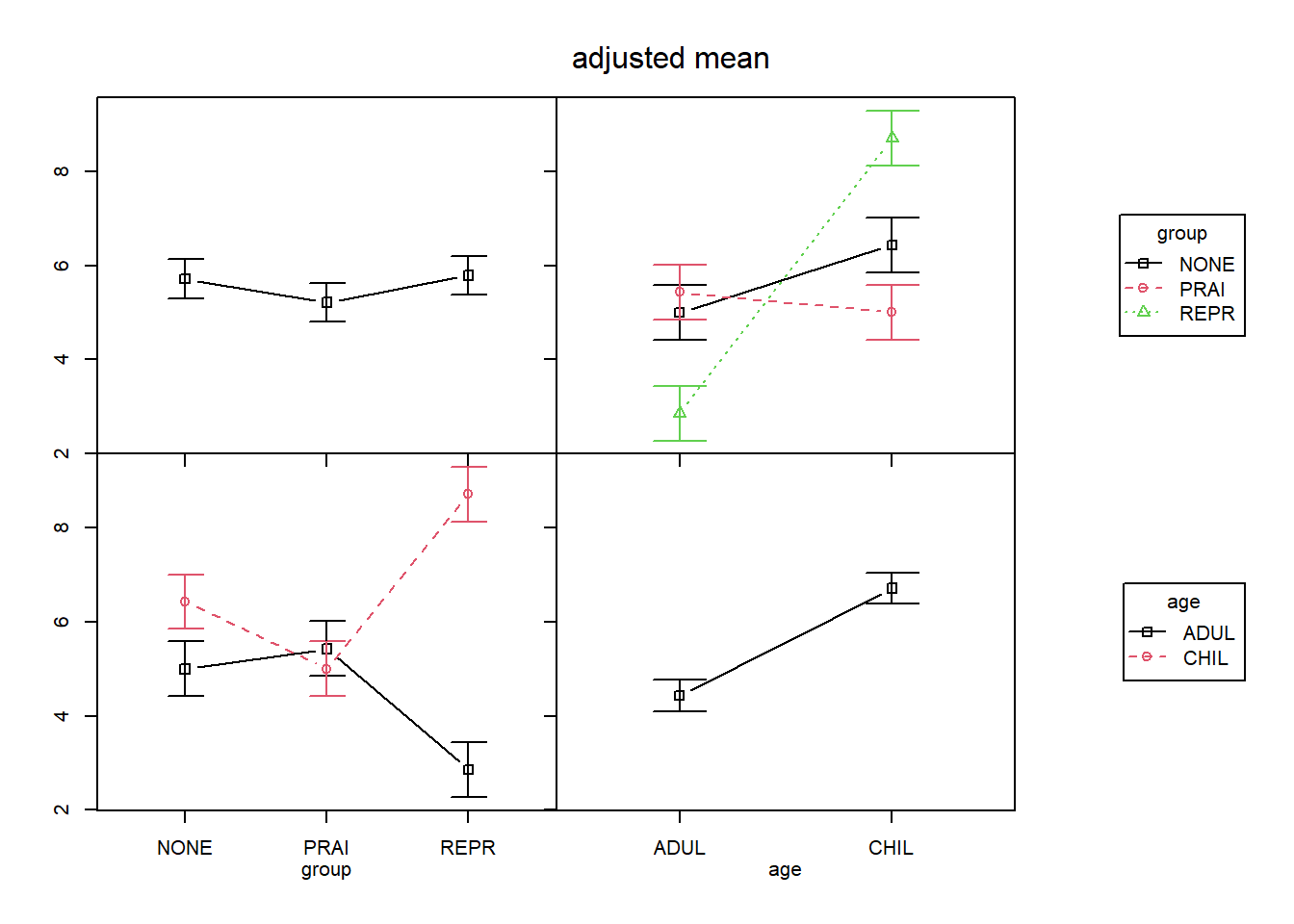We can also use the ‘interactionMeans’ function to obtain marginal means.

``````# obtain the marginal means for the two IVs (The main effect means)
# NOTE: the marginal means produced by interactionMeans appear to be
# UNWEIGHTED marginal means, when the design is unbalanced (unequal N)
interactionMeans(fit.2aov, factors="group")``````
``````##     group adjusted mean std. error
## 1  PRAISE      5.214286   0.412393
## 2 REPROOF      5.785714   0.412393
## 3    NONE      5.714286   0.412393``````
``interactionMeans(fit.2aov, factors="age")``
``````##        age adjusted mean std. error
## 1 CHILDREN      6.714286  0.3367175

## 6.1 Use of the `testInteractions` function in phia

The `testInteractions` function is very powerful, but not intuitive in its argument specifications.

It yields a table of output that does have easily recognized df,SS and F values with p values. The column in the table that is labeled “Value” is useful. If we use orthogonal contrasts (as we have done here), then the “Value” is our linear combination quantity that we have called PSI. If the default coding is used, then the “Values” are cell mean differences as would follow from dummy coding on that variable. In our particular example the rows are labeled clearly except that when using contrasts, an effect labeled “group1”, for example, is the first contrast on the “group” factor.

Now we are ready to obtain all of the effects of interest.
We want to obtain tests of main effect contrasts, interaction contrasts, simple main effects and their contrasts.

Note that the `testInteractions` function has an argument titled “adjustment” that can apply p-value adjustments (e.g., Sidak, etc) if needed. Those are not illustrated here.

### 6.1.1 Main effect and interaction contrasts

``````## NOTE: **phia** requires the contrasts in a different format than we used above
## ALSO NOTE: this produces the same SS and F's as the summary/split approach above

# create the contrasts on the treatment group factor and make them usable objects
ac1 <- list(group=c(-.5, 1, -.5)) # define first contrast on factor A which is group
ac2 <- list(group=c(1,0,-1))  # define second contrast on factor A which is group``````

This code chunk begins the analyses available from phia by evaluating the two main effect contrasts for the grouping factor, using the contrasts defined as “ac1” and “ac2”. These would not be of interest in the “real” analysis of this data set because there is an interaction present and because the main effect was not significant (mostly because of the former). It is included here so that the template is in place if it is needed for other data sets that use this same code sequence.

The adjustment argument permits specification of a member of the bonferroni family of error rate corrections if desired.

``````# First, main effect contrasts on group.
# These will be will be type III SS if unequal N
# Recall that in this study the main effect SS for the treatment group
# was very small since the three means were close together.  Note that the
# SS for these contrasts add up to that SS for the main effect of
# treatment group (2.714)
``````## F Test:
##             Value Df Sum of Sq     F Pr(>F)
## group1    0.32143  1     0.964 0.405 0.5285
## Residuals         36    85.714``````
``testInteractions(fit.2aov, custom=ac2, adjustment="none")``
``````## F Test:
##           Value Df Sum of Sq     F Pr(>F)
## group1     -0.5  1     1.750 0.735 0.3969
## Residuals       36    85.714``````

Note that adding the SS for the two contrasts yields the value of the SS for the main effect of group obtained above with the omnibus analyses.

``.964+1.750``
``##  2.714``

Next, we can obtain the interaction contrasts. Since “group” had two contrasts, we request a test with each of them separately. Notice that the interaction is produced by specifying the “across” argument with the other factor.

``````# interaction contrasts.  will be type III SS if unequal N
# do their SS sum to what they should?
``````## F Test:
##            Value Df Sum of Sq      F   Pr(>F)
## group1    5.3571  1    66.964 28.125 5.93e-06 ***
## Residuals        36    85.714
## ---
## Signif. codes:  0 '***' 0.001 '**' 0.01 '*' 0.05 '.' 0.1 ' ' 1``````
``testInteractions(fit.2aov, custom=ac2, adjustment="none", across="age")``
``````## F Test:
##             Value Df Sum of Sq     F Pr(>F)
## group1    -1.8571  1     6.036 2.535 0.1201
## Residuals         36    85.714``````

The sum of the SS for these two interaction contrasts produces the same value for SS of the 2 df interaction term seen above.

``66.964+6.036``
``##  73``

### 6.1.2 Simple main effects and simple main effect contrasts

Simple main effects are obtained with the same phiafunction, while passing different arguments to set the level of the factor held constant. In this case I have first asked for the simple effects of group at levels of age by using the “fixed” argument for age. The second requests the other set by fixing group.

Since “group” has three levels, each SME has two df. The error df reflect the fact that these tests each use the overall MSwg error term. Each SME of age at levels of group has 1 df since there are only two levels of age.

``````# now obtain simple main effects of group at levels of age
``````## F Test:
##            group1   group2 Df Sum of Sq     F    Pr(>F)
## CHILDREN   3.0000 -1.42857  2    49.143 10.32 0.0002866 ***
##   ADULTS  -2.3571  0.42857  2    26.571  5.58 0.0077467 **
## Residuals                  36    85.714
## ---
## Signif. codes:  0 '***' 0.001 '**' 0.01 '*' 0.05 '.' 0.1 ' ' 1``````
``````# and now simple main effects of age at levels of group
``````## F Test:
##             Value Df Sum of Sq     F    Pr(>F)
##  PRAISE   -0.4286  1     0.643  0.27   0.60651
## REPROOF    5.8571  1   120.071 50.43 2.418e-08 ***
##    NONE    1.4286  1     7.143  3.00   0.09183 .
## Residuals         36    85.714
## ---
## Signif. codes:  0 '***' 0.001 '**' 0.01 '*' 0.05 '.' 0.1 ' ' 1``````

We can double check to see that the sum of the SS for this set of SME adds up to what it should. It should equal the pooling of the SS for the main effect of group and the interaction (just as the 2+2 df pooling equals the pooling of the df for the main effect of group and the interaction).

``49.143+26.571``
``##  75.714``

Finally, we can partition the SME of group at levels of age into their single df contrasts using the same contrasts already specified.

``````# now obtain sme contrasts for the group factor
``````## F Test:
##                     Value Df Sum of Sq     F    Pr(>F)
## CHILDREN : group1  3.0000  1    42.000 17.64 0.0001675 ***
##   ADULTS : group1 -2.3571  1    25.929 10.89 0.0021865 **
## Residuals                 36    85.714
## ---
## Signif. codes:  0 '***' 0.001 '**' 0.01 '*' 0.05 '.' 0.1 ' ' 1``````
``testInteractions(fit.2aov, custom=ac2, adjustment="none", fixed="age")``
``````## F Test:
##                      Value Df Sum of Sq    F  Pr(>F)
## CHILDREN : group1 -1.42857  1     7.143 3.00 0.09183 .
##   ADULTS : group1  0.42857  1     0.643 0.27 0.60651
## Residuals                  36    85.714
## ---
## Signif. codes:  0 '***' 0.001 '**' 0.01 '*' 0.05 '.' 0.1 ' ' 1``````

The conclusion here is that the ‘testInteractions’ function is very flexible. All F tests match what we obtained in manual illustrations and with SPSS’ MANOVA analyses.

# 7 Using emmeans for examination of followup effects

The emmeans package provides an alternate facility for performing follow up analyses to the omnibus effects in factorial designs. It’s general logic is to set a grid of descriptive stats for the groups in the design and then perform analyses using that grid. P value adjustments can also be applied to effects evaluated with emmeans. Some of those are illustrated in this section and others in the PostHoc/MultipleComparisions section that follows.

## 7.1 Extract the grid of means to work with

We can us the original `aov` model object used above to produce the omnibus analyses. Note that `emmeans` also produces a test of the global omnibus effect of groups - treating the factorial as a 1-way design with six groups…….probably not an interesting inference.

A caveat: This section used the fit.2aov object where the orthogonal contrasts were employed. But it doesn’t matter which object is employed since emmeans extracts only the means and the error term MS to use in it’s inferences. We could have used the dummy-coded or effect-coded objects as well. But when it comes to unbalanced designs and marginal effects such as main effects, it is best to begin with the object that used the orthogonal contrasts. In fact, follow up analyses with dummy-coded object is not recommended for factorials.

``````#https://cran.r-project.org/web/packages/emmeans/vignettes/interactions.html
fit1.emm <- emmeans(fit.2aov, c("group","age"))
fit1.emm``````
``````##  group   age      emmean    SE df lower.CL upper.CL
##  PRAISE  CHILDREN   5.00 0.583 36     3.82     6.18
##  REPROOF CHILDREN   8.71 0.583 36     7.53     9.90
##  NONE    CHILDREN   6.43 0.583 36     5.25     7.61
##  PRAISE  ADULTS     5.43 0.583 36     4.25     6.61
##  REPROOF ADULTS     2.86 0.583 36     1.67     4.04
##  NONE    ADULTS     5.00 0.583 36     3.82     6.18
##
## Confidence level used: 0.95``````
``test(contrast(fit1.emm, "eff"), joint=TRUE)``
``````##  df1 df2 F.ratio p.value note
##    5  36  10.968  <.0001  d
##
## d: df1 reduced due to linear dependence``````

## 7.2 Simple main effects with `emmeans`

Simple Main Effects of group at levels of age are found by establishing a matrix of means involving the group factor at levels of age. ‘emmeans’ permits this specification with the “by” argument and the inferential tests for each of the two tables are, in fact, the 2 df simple main effects, and the results match what we saw above with phia and with our SPSS work.

``````fit1.emm.g <- emmeans(fit.2aov, "group", by="age")
fit1.emm.g``````
``````## age = CHILDREN:
##  group   emmean    SE df lower.CL upper.CL
##  PRAISE    5.00 0.583 36     3.82     6.18
##  REPROOF   8.71 0.583 36     7.53     9.90
##  NONE      6.43 0.583 36     5.25     7.61
##
##  group   emmean    SE df lower.CL upper.CL
##  PRAISE    5.43 0.583 36     4.25     6.61
##  REPROOF   2.86 0.583 36     1.67     4.04
##  NONE      5.00 0.583 36     3.82     6.18
##
## Confidence level used: 0.95``````
``test(contrast(fit1.emm.g, "eff"), joint=TRUE)``
``````##  age      df1 df2 F.ratio p.value note
##  CHILDREN   2  36  10.320  0.0003  d
##  ADULTS     2  36   5.580  0.0077  d
##
## d: df1 reduced due to linear dependence``````

Simple Main Effect contrasts require passing a list of those explicit contrasts to the contrast function. It works here because these contrasts are applied in the context of the “fit1.emm.g” object which already structured the effects as the effects of group at levels of age. Note that the tests employ t statistics. Squaring them gives the F’s we have found elsewhere.

``````lincombs_gsme <- contrast(fit1.emm.g,
list(ac1=c(-.5,1, -.5), ac2=c(1,0,-1))) # second one not changed
``````## age = CHILDREN:
##  contrast estimate    SE df t.ratio p.value
##  ac1         3.000 0.714 36   4.200  0.0002
##  ac2        -1.429 0.825 36  -1.732  0.0918
##
##  contrast estimate    SE df t.ratio p.value
##  ac1        -2.357 0.714 36  -3.300  0.0022
##  ac2         0.429 0.825 36   0.520  0.6065``````

## 7.3 Main effect contrasts with `emmeans`

We can also create an emm object for the main effect means; in this case the main effect of group marginal means are requested. The ‘pairs’ function permits pairwise comparison among the set of requested means. This pairwise comparison might be done using Tukey tests, as was done here, or other adjustments (“none” would give no correction for alpha inflation). One could use this pairs approach on any matrix of means derived by the ‘emmeans’ function. Note that we would probably not be interested in these main effect comparisons here because of the presence of an interaction.

``fit1.emm.a <- emmeans(fit.1aov, "group", data=prn.data)``
``## NOTE: Results may be misleading due to involvement in interactions``
``pairs(fit1.emm.a, adjust="tukey")``
``````##  contrast         estimate    SE df t.ratio p.value
##  PRAISE - REPROOF  -0.5714 0.583 36  -0.980  0.5942
##  PRAISE - NONE     -0.5000 0.583 36  -0.857  0.6703
##  REPROOF - NONE     0.0714 0.583 36   0.122  0.9918
##
## Results are averaged over the levels of: age
## P value adjustment: tukey method for comparing a family of 3 estimates``````

We can also obtain main effect contrasts for the group factor in an analogous manner.

``````lincombs_g <- contrast(fit1.emm.a,
list(ac1=c(-.5,1, -.5), ac2=c(1,0,-1))) # second one not changed
``````##  contrast estimate    SE df t.ratio p.value
##  ac1         0.321 0.505 36   0.636  0.5285
##  ac2        -0.500 0.583 36  -0.857  0.3969
##
## Results are averaged over the levels of: age``````

## 7.4 Interaction contrasts with `emmeans`

Exploring interaction contrasts with `emmeans` involves a bit more effort. Since all of the means in the factorial are involved in interactions, we work with the grid of six means initially established above and called “fit1.emm”. In order to find the two interaction contrasts (the interaction has 2 df to be decomposed into single df terms) we need to construct contrast vectors that have six coefficients, one for each group. Please recall that we have done this elsewhere and this table should remind you of that: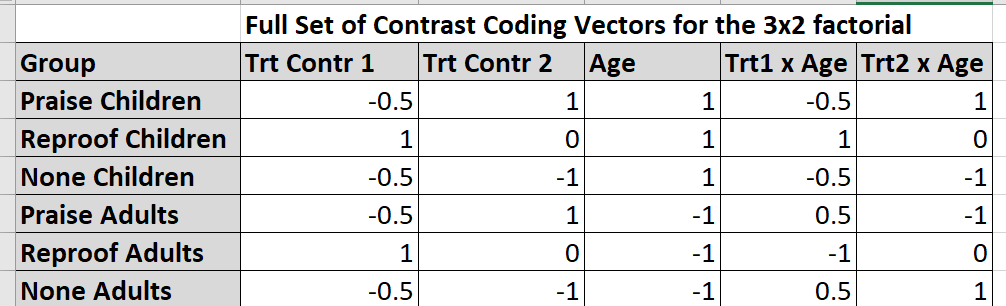Now that we have the two coefficient vectors for the interaction contrasts, we can apply them to the six-group grid of means. Notice that the order of the coefficients is important relative to the ordering of the groups in the “fit1.emm” object. This is why comparison of those means in the fit1.emm grid and the coefficients to the ordering seen in the table just above is important. The analysis reproduces estimates and t values first see in use of the `summary.lm` function on the fit.2aov object and their squares match the F’s seen with the comparable phia tests (within rounding error taking these t’s to three decimal places).

``````lincombs_int <- contrast(fit1.emm,
list(intc1=c(-.5,1, -.5, .5, -1, .5),
intc2=c(1,0,-1, -1,0,1)))
``````##  contrast estimate   SE df t.ratio p.value
##  intc1        5.36 1.01 36   5.303  <.0001
##  intc2       -1.86 1.17 36  -1.592  0.1201``````

Note that we had, perhaps more efficiently, obtained these interaction contrasts in an earlier section where we used the `summary.lm` function on the `aov` fit object. The `testInteractions` function in phia also provided a straight forward way to obtain them. I prefer a mix of using these three approaches.

# 8 Post Hoc and Multiple Comparison Approaches

Some capability for adjustment of p values for the multiple testing situation was demonstrated in the phia and emmeans sections above. The p value adjustments are especially valuable if the user needs their application in the use of contrasts. The pairwise comparison methods are typically called Multiple Comparisons. The family of these is large. This section focuses on use of the Tukey test in part because that is the most visibly apparent in the R ecosystem and in part because application of these methods is a logical challenge in factorial designs.

Consider the simple 3x2 design illustrated above in this document. There are six cells in the factorial. Control of Type I error rate inflation might consider controlling for comparisons of all possible pairs of means…… six means, therefore 15 possible pairs to compare. But not all of thes pairs will be of interest. Specifically comparisons that change levels of both factors simultaneously are not typically interesting and therefore, alpha rate control may not be necessary. This topic has been discussed by Cichetti as seen on our class work. The interesting pairwise comparisons are either within rows or within columns of the design or among one or both sets of marginals. These comprise different families of comparisons and one strategy is to control the alpha rate inflation separately within each family. The reader will recognize that comparisons within rows or within columns would be a way to follow up simple main effects described above.

This section begins with the possible use of the Tukey HSD test. Tukey’s HSD is easily accomplished on an AOV fit via a function in the base stats package that is loaded upon startup. Note that this compares all pairs of marginals and all pairs of cell means. it would severely over correct for alpha inflation with the cell means pairs since not all of the pairs of cell means are interesting comparisons.

Application of all the other post hoc comparison methods (e.g., Dunnett, REGWQ, etc) within various “families” is problematic for direct approaches to factorial designs in R. Manual computation may be the best solution, although we will return to a general strategy later once higher order factorials are covered.

The overkill “all possible pairs” approach using ‘TukeyHSD’ is shown here, although not recommended, especially for the fifteen pairwise comparisons among all six cells. Of these the only intersting comparisons would be within rows or columns. That yields a set of only nine. But the overkill comes from the fact that the TukeyHSD adjustment presumes all 15 should b